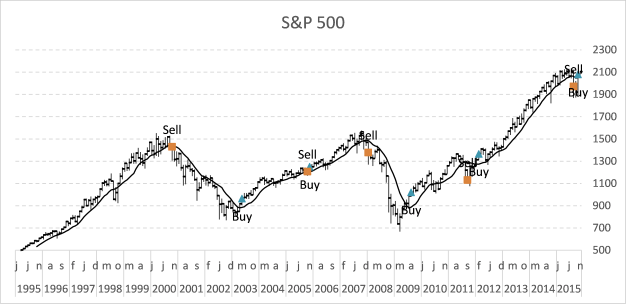Author: Oscar Cronquist Article last updated on August 06, 2017

In my previous post I described how to build a dynamic stock chart that lets you easily adjust the date range and change index/company. Price data is quickly and automatically fetched from yahoo finance.

This post shows you how to add tools to the charts so you can quickly identify the trend for a stock or index. The stock market often trends for many months up or down and a moving average smooths out price data.

The examples shown in this post are based on S&P 500 index and larger trends. Charts show price data on a monthly scale and the date range is from 1995 to present time.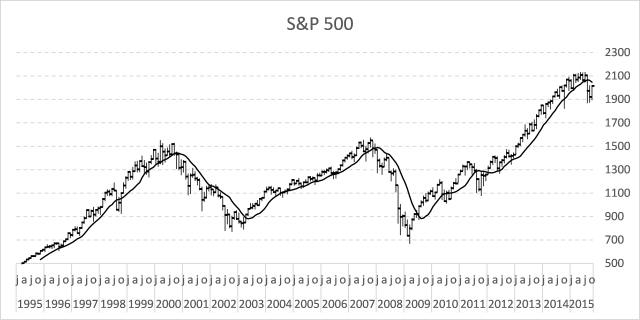This excel stock chart has a 10 month moving average. It can be calculated using the AVERAGE function on an excel worksheet.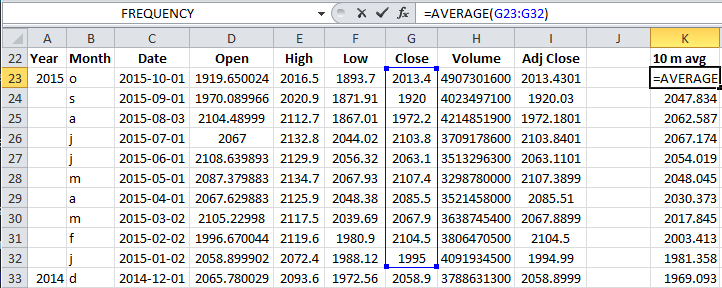Formula in cell K23:

=AVERAGE(G24:G33)

This function calculates the average from 10 latest closing prices. Copy cell K23 and paste to cells below as far as needed.

### How to add a moving average to an excel stock chart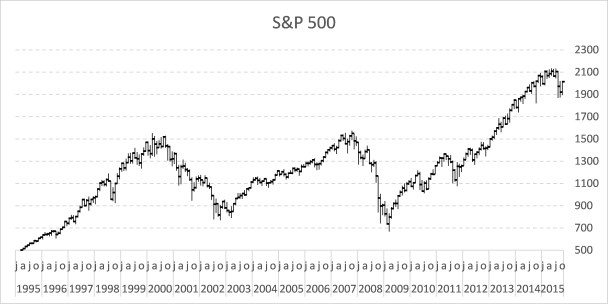1. Right click on chart
2. Click on "Select Data..."
4. Select cell range \$K\$23:\$K\$2725. Click OK button
6. Go to tab "Layout" on the ribbon.
7. Select "Series 4"8. Click "Format Selection" button, see picture above.
9. Select "Secondary Axis"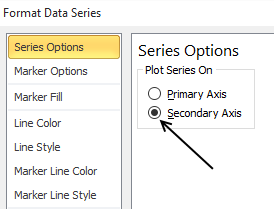10. Go to "Line Color"
11. Select "Solid Line"
12. Pick a color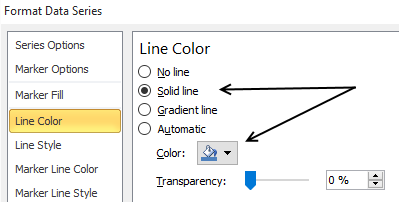The chart now looks like this: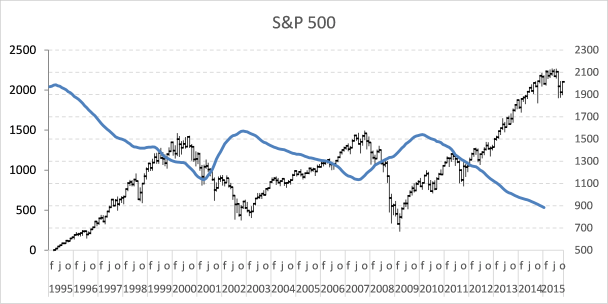The data on the secondary axis has to be reversed.

1. Select line on chart
2. Go to tab "Layout" on the ribbon
3. Click "Axis" button and then "Secondary horizontal axis" and finally click "Show right to left axis"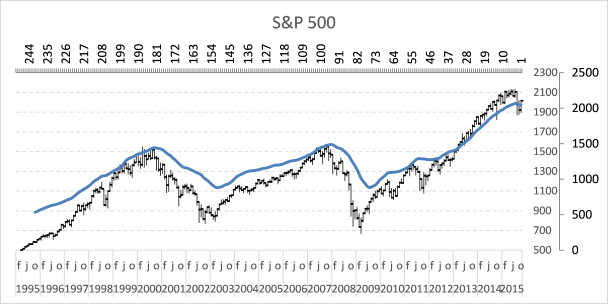4. Select and delete the horizontal axis above the chart
5. Go to tab "Layout" on the ribbon
6. Click "Axis" button and "Secondary Vertical Axis" and finally "None"The chart looks like this: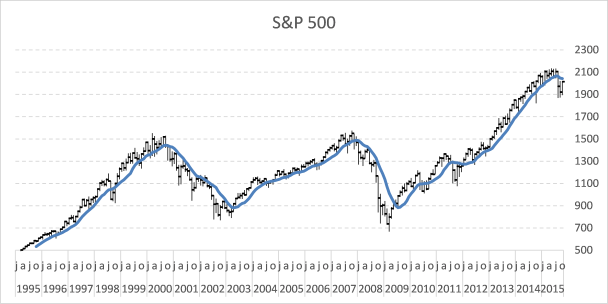A moving average indicates if a market is about to go up or down in the long term.

### Plot moving average turning points

Let's start with calculating when the moving average changes from moving down to up.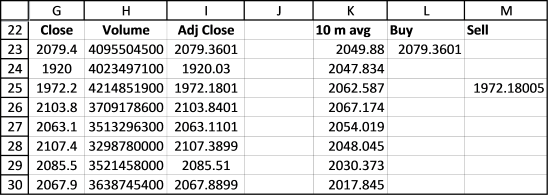=IF((K23>K24)*(K24<K25),G23,"")

If the value in cell K23 is larger than cell K24 AND cell K24 is smaller than K25 THEN return the closing price.  If not return nothing.

The next formula calculates when the moving average changes from going up to going down.

Sell formula in cell M23:

=IF((K23<K24)*(K24>K25),G23,"")

Copy cell range L23:M23 and paste to cells below as far as needed. It is now time to plot these moving average turning points.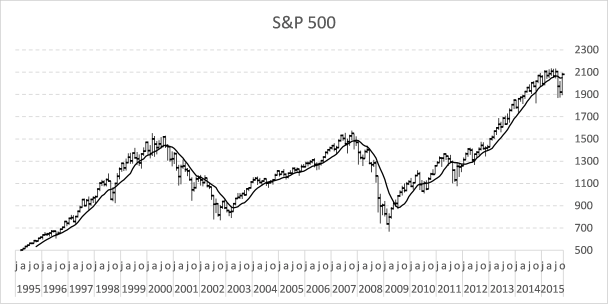1. Right click on chart
2. Click "Select Data..."
4. Select cell range \$L\$23:\$L\$273
5. Click OK button
7. Select cell range \$M\$23:\$M\$273
8. Click OK button
9. Click OK button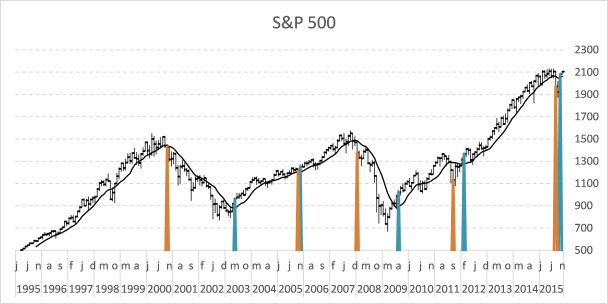Here is how to remove lines shown above and use markers instead.

1. Go to tab "Layout"
2. Select Series 5
3. Click "Format Selection"
4. Select "Marker Options"
5. Select "Built-in"
6. Pick a type
7. Go to "Line Color"
8. Select "No Line"
9. Select "Series 6" on tab "Layout" on the ribbon
10. Select "Marker Options"
11. Select "Built-in"
12. Pick a type
13. Go to "Line Color"
14. Select "No Line"### How to extract the moving average turning dates using excel array formulas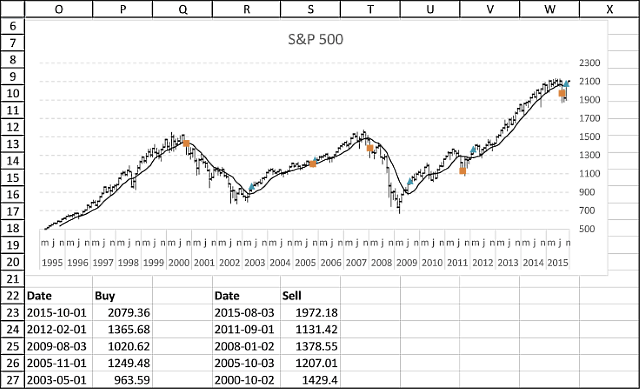Array formula (Buy) in cell P23:

=IFERROR(INDEX(\$G\$23:\$G\$262, SMALL(IF((\$K\$23:\$K\$262>\$K\$24:\$K\$263)*(\$K\$24:\$K\$263<\$K\$25:\$K\$264), MATCH(ROW(\$G\$23:\$G\$262), ROW(\$G\$23:\$G\$262)), ""), ROWS(\$A\$1:A1))), "")

Formula (Buy Date) in cell O23:

=IFERROR(INDEX(\$C\$23:\$C\$262,MATCH(P23,\$G\$23:\$G\$262,0)),"")

Array formula (Sell) in cell S23:

=IFERROR(INDEX(\$G\$23:\$G\$262, SMALL(IF((\$K\$23:\$K\$262<\$K\$24:\$K\$263)*(\$K\$24:\$K\$263>\$K\$25:\$K\$264), MATCH(ROW(\$G\$23:\$G\$262), ROW(\$G\$23:\$G\$262)), ""), ROWS(\$A\$1:A1))), "")

Formula (Sell Date) in cell R23:

=IFERROR(INDEX(\$C\$23:\$C\$262,MATCH(S23,\$G\$23:\$G\$262,0)),"")

How to enter an array formula

1. Select cell P23
2. Copy array formula
3. Paste array formula in formula bar
4. Press and hold CTRL + SHIFT simultaneously
5. Press Enter once
6. Release all keys

If you did the above instructions correctly, the formula begins and ends with a curly bracket, like this {=formula}. Don´t enter these characters yourself.

Make sure you enter array formulas in cell P23 and S23. Then copy cell P23 and paste to cells below as far as needed. Repeat with cell S23, R23 and O23.